# Test Prep Plan - Take a practice test

Take this practice test to check your existing knowledge of the course material. We'll review your answers and create a Test Prep Plan for you based on your results.
How Test Prep Plans work
1
2Based on your results, we'll create a customized Test Prep Plan just for you!
3Study smarter
Study more effectively: skip concepts you already know and focus on what you still need to learn.

# NMTA Math: Circles & Arc of a Circle Chapter Exam

Exam Instructions:

Choose your answers to the questions and click 'Next' to see the next set of questions. You can skip questions if you would like and come back to them later with the yellow "Go To First Skipped Question" button. When you have completed the practice exam, a green submit button will appear. Click it to see your results. Good luck!

### Page 1

#### Question 5 5. In the picture below, if AE is 10, AB is 14 and ED is 16, what is the length of CE?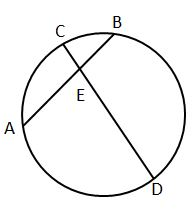### Page 2

#### Question 7 7. In the picture below, if AC is 14 and AB is 10, what is the length of AD?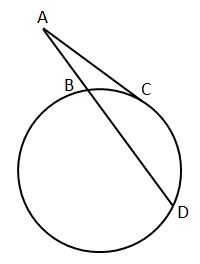#### Question 10 10. In the picture below, if major arc BC is 258 degrees and minor arc BC is 102 degrees, what is the measure of angle BAC?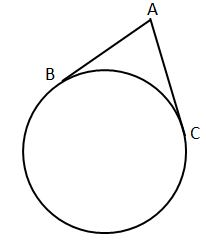### Page 3

#### Question 11 11. If arc AB is 72 degrees, what is the measure of angle ACB?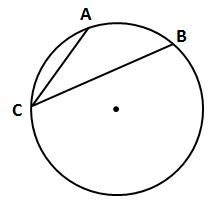### Page 4

#### Question 16 16. If arc AC is 106 degrees and arc BC is 82 degrees, what is the measure of angle ACB?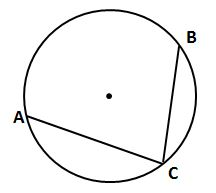#### Question 17 17. In the picture below, if arc AB is 82 degrees, what is the measure of angle BAC?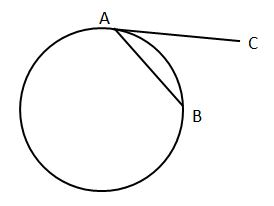#### Question 18 18. In the picture below, if AB is 7, BC is 19 and AD is 8, what is the length of DE?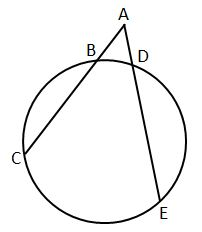#### Question 19 19. If angle OAB is 45 degrees, what is the measure of angle AOB?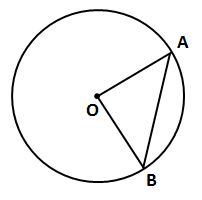#### Question 20 20. If angle ACB is 34 degrees, what is the measure of angle ADB?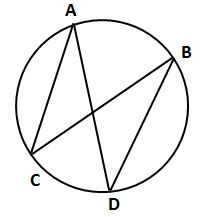### Page 5

#### Question 24 24. In the picture below, if arc AC is 146 degrees and arc BD is 32 degrees, what is the measure of angle AEC?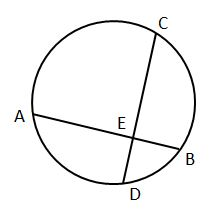### Page 6

#### Question 26 26. If angle ACB is 47 degrees, what is the measure of angle AOB?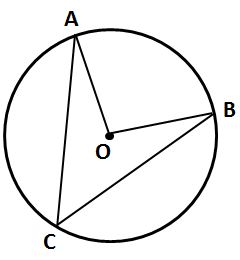#### Question 27 27. If AB and CB are tangent segments, what is the length of CB?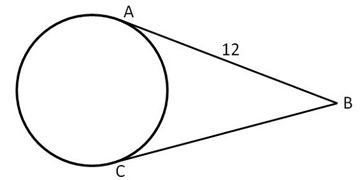#### Question 28 28. If angle AOB is 40 degrees, what is the measure of angle ACB?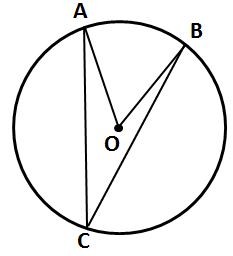#### NMTA Math: Circles & Arc of a Circle Chapter Exam Instructions

Choose your answers to the questions and click 'Next' to see the next set of questions. You can skip questions if you would like and come back to them later with the yellow "Go To First Skipped Question" button. When you have completed the practice exam, a green submit button will appear. Click it to see your results. Good luck!

Support